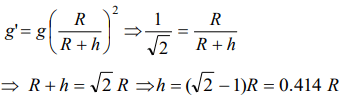## Gravitation Questions and Answers Part-8

1. The height of the point vertically above the earth’s surface, at which acceleration due to gravity becomes 1% of its value at the surface is (Radius of the earth = R)
a) 8 R
b) 9 R
c) 10 R
d) 20 R

Explanation: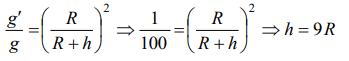2. An object weights 72 N on earth. Its weight at a height of R/2 from earth is
a) 32 N
b) 56 N
c) 72 N
d) Zero

Explanation: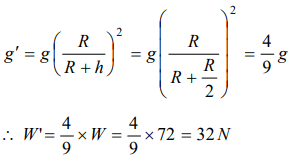3. The angular velocity of the earth with which it has to rotate so that acceleration due to gravity on 60o latitude becomes zero is (Radius of earth = 6400 km. At the poles $g=10ms^{-2 }$
a) $2.5\times10^{-3 }rad\diagup s$
b) $5.0\times10^{-1 }rad\diagup s$
c) $10\times10^{1 }rad\diagup s$
d) $7.8\times10^{-2 }rad\diagup s$

Explanation: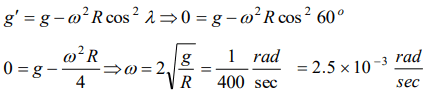4. Assuming earth to be a sphere of a uniform density, what is the value of gravitational acceleration in a mine 100 km below the earth’s surface (Given R = 6400 km)
a) 9.66 $m\diagup s^{2 }$
b) 7.64 $m\diagup s^{2 }$
c) 5.06 $m\diagup s^{2 }$
d) 3.10 $m\diagup s^{2 }$

Explanation:5. If radius of earth is R then the height ‘h’ at which value of ‘g’ becomes one-fourth is
a) $\frac{R}{4}$
b) $\frac{3R}{4}$
c) R
d) $\frac{R}{8}$

Explanation:6. R and r are the radii of the earth and moon respectively. $\rho_{e}$ and $\rho_{m}$ are the densities of earth and moon respectively. The ratio of the accelerations due to gravity on the surfaces of earth and moon is
a) $\frac{R}{r}\frac{\rho_{e}}{\rho_{m}}$
b) $\frac{r}{R}\frac{\rho_{e}}{\rho_{m}}$
c) $\frac{r}{R}\frac{\rho_{m}}{\rho_{e}}$
d) $\frac{R}{r}\frac{\rho_{m}}{\rho_{e}}$

Explanation:7. If the mass of earth is 80 times of that of a planet and diameter is double that of planet and ‘g’ on earth is $9.8 m\diagup s^{2 }$ , then the value of ‘g’ on that planet is
a) 4.9 $m\diagup s^{2 }$
b) 0.98 $m\diagup s^{2 }$
c) 0.49 $m\diagup s^{2 }$
d) 49 $m\diagup s^{2 }$

Explanation: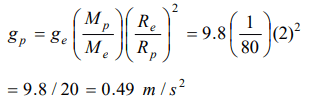8.Assume that the acceleration due to gravity on the surface of the moon is 0.2 times the acceleration due to gravity on the surface of the earth. If Re is the maximum range of a projectile on the earth’s surface, what is the maximum range on the surface of the moon for the same velocity of projection
a) $0.2R_{e}$
b) $2 R_{e}$
c) $0.5 R_{e}$
d) $5 R_{e}$

Explanation:9. The angular speed of earth, so that the object on equator may appear weightless, is (g = 10 m/s2 , radius of earth 6400 km)
a) $1.25\times 10^{-3}rad/sec$
b) $1.56\times 10^{-3}rad/sec$
c) $1.25\times 10^{-1}rad/sec$
d) 1.56 $rad/sec$